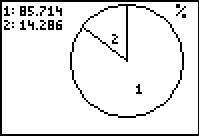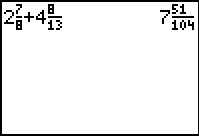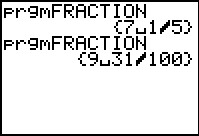# Activities

••• ##### Subject Area

• Math: Middle Grades Math: Algebraic Thinking
• Math: Middle Grades Math: Number Sense

• ##### Author6-8

45 Minutes

• ##### Device
• TI-73 Explorer™
• TI-Navigator™
• ##### Software

LearningCheck™ Creator
TI Connect™

• ##### Accessories

TI Connectivity Cable

## Estimation Station#### Activity Overview

In this activity, students will use a Pie Chart to help them round fractions and mixed numbers. Then they will use their rounding skills to estimate sums and differences.

#### Key Steps

•In the first problem, students will use a pie chart to help them determine whether to round a fraction to the nearest whole number or nearest half. If section 1 takes up most or none of the circle students will round to the nearest whole, and if the section 1 takes up only half of the circle, they will round to the nearest half.

•In Problems 2 and 3, students will use the rounding skills they learned in the previous problem to estimate sums and differences.

•As an extension or homework assignment, students can use a program to generate random fractions to use in addition and subtraction problems. Again, they will use rounding to estimate solutions before finding exact answers.